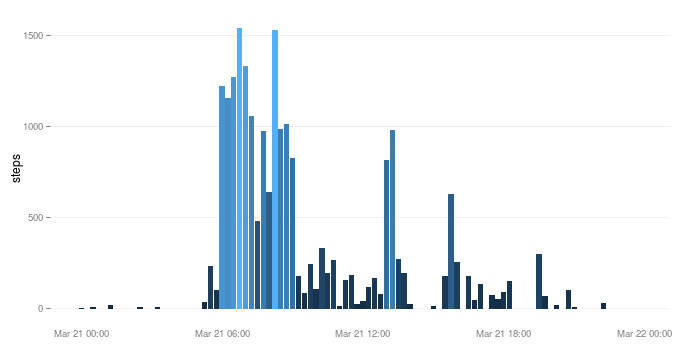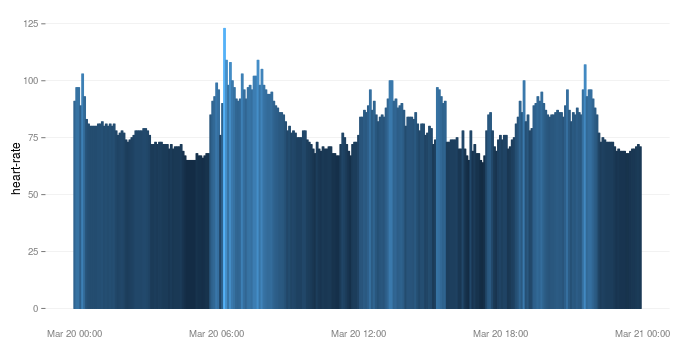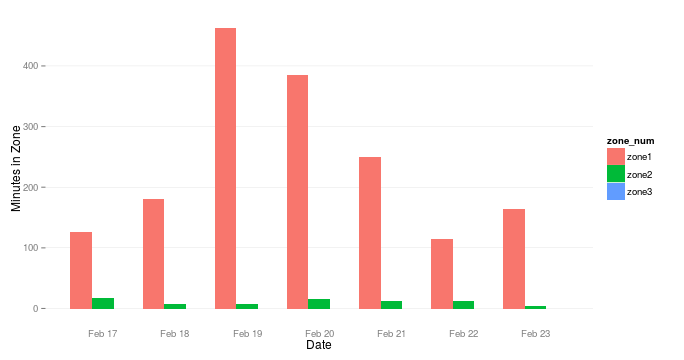## Thursday, March 26, 2015

### fitbitScraper 0.1.2 Now on CRAN

I've updated the fitbitScraper to 0.1.2 with the help of a friend that gave me access to his fitbit account so I could work out the heart rate API endpoints (thanks Shane!). This is a package that enables you to read daily or intraday data from Fitbit into R for graphing and analysis.

Here's some examples:

I'll download all my steps for last Saturday, March 21, 2015, then make a graph similar to how it appears on the fitbit dashboard. As you can see, I spent some time playing basketball in the morning and pretty much took it easy the rest of the day. Note that the function name has changed to get_intraday_data() from get_15_min_data() in version 0.1.1.

``````# install.packages("fitbitScraper")
library("fitbitScraper")
# just reading from file to hide pw and to make .Rmd document to work...
library("ggplot2")
ggplot(df) + geom_bar(aes(x=time, y=steps, fill=steps), stat="identity") +
xlab("") +ylab("steps") +
theme(axis.ticks.x=element_blank(),
panel.grid.major.x = element_blank(),
panel.grid.minor.x = element_blank(),
panel.grid.minor.y = element_blank(),
panel.background=element_blank(),
panel.grid.major.y=element_line(colour="gray", size=.1),
legend.position="none")
``````Now, let's take a look at the heart rate data. This is Shane's data… I have no idea what he was doing on this day. Note that whereas intraday data for steps is given in 15 minute intervals, fitbit provides the heart rate data in 5 minute intervals.

``````# just reading from file to hide pw and to make .Rmd document to work...
library("ggplot2")
ggplot(df) + geom_bar(aes(x=time, y=`heart-rate`, fill=`heart-rate`,
colour=`heart-rate`), stat="identity") +
xlab("") +ylab("heart-rate") +
theme(axis.ticks.x=element_blank(),
panel.grid.major.x = element_blank(),
panel.grid.minor.x = element_blank(),
panel.grid.minor.y = element_blank(),
panel.background=element_blank(),
panel.grid.major.y=element_line(colour="gray", size=.1),
legend.position="none")
``````And finally, the daily heart rate data comes with three data points for each day. Zone1, zone2, and zone3 presumably represent the time in a given heart rate zone. I suspect they correspond to “fat burn zone”, “cardio zone”, and “peak zone” respectively. More information on fitbit heart rate zones is available here.

``````# just reading from file to hide pw and to make .Rmd document to work...
start_date="2015-02-17", end_date="2015-02-23")
library("reshape")
df <- melt(df, id="time", variable_name="zone_num")
library("ggplot2")
ggplot(df) + geom_bar(aes(x=time, y=value, fill=zone_num),
position=position_dodge(), stat="identity") +
xlab("Date") +ylab("Minutes in Zone") +
theme(axis.ticks.x=element_blank(),
panel.grid.major.x = element_blank(),
panel.grid.minor.x = element_blank(),
panel.grid.minor.y = element_blank(),
panel.background=element_blank(),
panel.grid.major.y=element_line(colour="gray", size=.1))
``````I'd love to work out the details for the GPS in the fitbit Surge. Unfortunately, I don't have one. If you are interested in working with me on integrating that data into the package, hit me up… corynissen@gmail.com